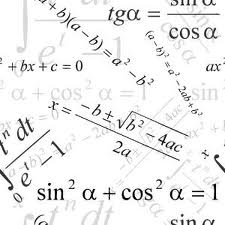# Always Divisible By 17$x$ and $y$ are integers such that $(2x+3y)$ is divisible by 17.

For which of the following values of $k$, must $9x + ky$ always be divisible by 17?

×